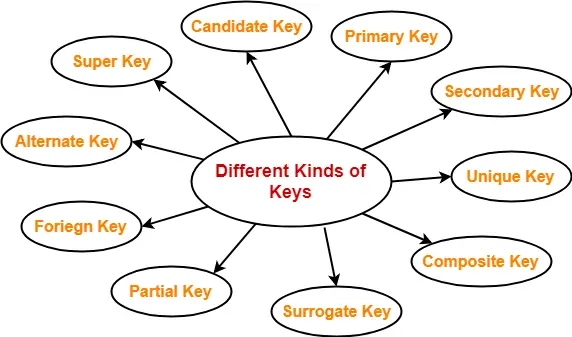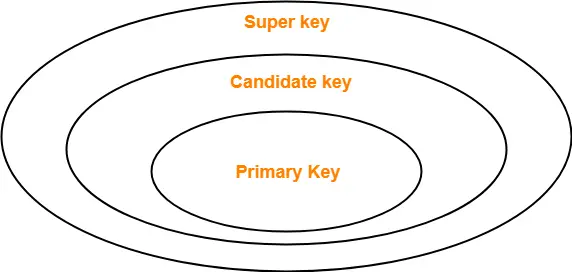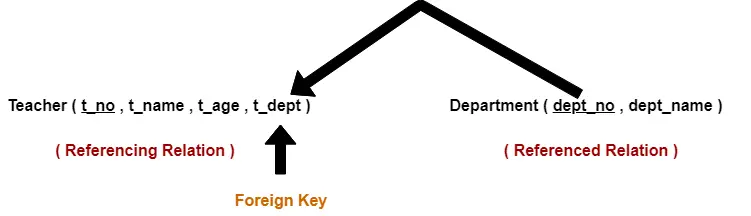## Keys in DBMS-

Before you go through this article, make sure that you have gone through the previous article on Keys in DBMS.

We have discussed-

• A key is a set of attributes that can identify each tuple uniquely in the given relation.
• There are various types of keys in DBMS-In this article, we will discuss how to find candidate keys of a given relation.

## Candidate Key-

A candidate key may be defined as-

 A set of minimal attribute(s) that can identify each tuple uniquely in the given relation is called as a candidate key.ORA minimal super key is called as a candidate key.

For any given relation,

• It is possible to have multiple candidate keys.
• There exists no general formula to find the total number of candidate keys of a given relation.

## Example-

Consider the following Student schema-

Student ( roll , name , sex , age , address , class , section )

Given below are the examples of candidate keys-

• ( class , section , roll )
• ( name , address )

These are candidate keys because each set consists of minimal attributes required to identify each student uniquely in the Student table.

## Finding Candidate Keys-

We can determine the candidate keys of a given relation using the following steps-

### Step-01:

• Determine all essential attributes of the given relation.
• Essential attributes are those attributes which are not present on RHS of any functional dependency.
• Essential attributes are always a part of every candidate key.
• This is because they can not be determined by other attributes.

### Example

Let R(A, B, C, D, E, F) be a relation scheme with the following functional dependencies-

A → B

C → D

D → E

Here, the attributes which are not present on RHS of any functional dependency are A, C and F.

So, essential attributes are- A, C and F.

### Step-02:

• The remaining attributes of the relation are non-essential attributes.
• This is because they can be determined by using essential attributes.

Now, following two cases are possible-

### Case-01:

If all essential attributes together can determine all remaining non-essential attributes, then-

• The combination of essential attributes is the candidate key.
• It is the only possible candidate key.

### Case-02:

If all essential attributes together can not determine all remaining non-essential attributes, then-

• The set of essential attributes and some non-essential attributes will be the candidate key(s).
• In this case, multiple candidate keys are possible.
• To find the candidate keys, we check different combinations of essential and non-essential attributes.

We will further understand how to find candidate keys with the help of following problems.

The following practice problems are based on Case-01.

## Problem-01:

Let R = (A, B, C, D, E, F) be a relation scheme with the following dependencies-

C → F

E → A

EC → D

A → B

Which of the following is a key for R?

1. CD
2. EC
3. AE
4. AC

Also, determine the total number of candidate keys and super keys.

## Solution-

We will find candidate keys of the given relation in the following steps-

### Step-01:

• Determine all essential attributes of the given relation.
• Essential attributes of the relation are- C and E.
• So, attributes C and E will definitely be a part of every candidate key.

Step-02:

Now,

• We will check if the essential attributes together can determine all remaining non-essential attributes.
• To check, we find the closure of CE.

So, we have-

{ CE }+

= { C , E }

= { C , E , F }                       ( Using C → F )

= { A , C , E , F }                  ( Using E → A )

= { A , C , D , E , F }            ( Using EC → D )

= { A , B , C , D , E , F }       ( Using A → B )

We conclude that CE can determine all the attributes of the given relation.

So, CE is the only possible candidate key of the relation.

Thus, Option (B) is correct.

### Total Number of Candidate Keys-

Only one candidate key CE is possible.

### Total Number of Super Keys-

There are total 6 attributes in the given relation of which-

• There are 2 essential attributes- C and E.
• Remaining 4 attributes are non-essential attributes.
• Essential attributes will be definitely present in every key.
• Non-essential attributes may or may not be taken in every super key.So, number of super keys possible = 2 x 2 x 2 x 2 = 16.

Thus, total number of super keys possible = 16.

## Problem-02:

Let R = (A, B, C, D, E) be a relation scheme with the following dependencies-

AB → C

C → D

B → E

Determine the total number of candidate keys and super keys.

## Solution-

We will find candidate keys of the given relation in the following steps-

### Step-01:

• Determine all essential attributes of the given relation.
• Essential attributes of the relation are- A and B.
• So, attributes A and B will definitely be a part of every candidate key.

Step-02:

Now,

• We will check if the essential attributes together can determine all remaining non-essential attributes.
• To check, we find the closure of AB.

So, we have-

{ AB }+

= { A , B }

= { A , B , C }                     ( Using AB → C )

= { A , B , C , D }               ( Using C → D )

= { A , B , C , D , E }          ( Using B → E )

We conclude that AB can determine all the attributes of the given relation.

Thus, AB is the only possible candidate key of the relation.

### Total Number of Candidate Keys-

Only one candidate key AB is possible.

### Total Number of Super Keys-

There are total 5 attributes in the given relation of which-

• There are 2 essential attributes- A and B.
• Remaining 3 attributes are non-essential attributes.
• Essential attributes will be definitely present in every key.
• Non-essential attributes may or may not be taken in every super key.So, number of super keys possible = 2 x 2 x 2 = 8.

Thus, total number of super keys possible = 8.

## Problem-03:

Consider the relation scheme R(E, F, G, H, I, J, K, L, M, N) and the set of functional dependencies-

{ E, F } → { G }

{ F } → { I , J }

{ E, H } → { K, L }

{ K } → { M }

{ L } → { N }

What is the key for R?

1. { E, F }
2. { E, F, H }
3. { E, F, H, K, L }
4. { E }

Also, determine the total number of candidate keys and super keys.

## Solution-

We will find candidate keys of the given relation in the following steps-

### Step-01:

• Determine all essential attributes of the given relation.
• Essential attributes of the relation are- E, F and H.
• So, attributes E, F and H will definitely be a part of every candidate key.

Step-02:

Now,

• We will check if the essential attributes together can determine all remaining non-essential attributes.
• To check, we find the closure of EFH.

So, we have-

{ EFH }+

= { E , F , H }

= { E , F , G , H }                                ( Using EF → G )

= { E , F , G , H , I , J }                       ( Using F → IJ )

= { E , F , G , H , I , J , K , L }             ( Using EH → KL )

= { E , F , G , H , I , J , K , L , M }       ( Using K → M )

= { E , F , G , H , I , J , K , L , M , N } ( Using L → N )

We conclude that EFH can determine all the attributes of the given relation.

So, EFH is the only possible candidate key of the relation.

Thus, Option (B) is correct.

### Total Number of Candidate Keys-

Only one candidate key EFH is possible.

### Total Number of Super Keys-

There are total 10 attributes in the given relation of which-

• There are 3 essential attributes- E, F and H.
• Remaining 7 attributes are non-essential attributes.
• Essential attributes will be definitely present in every key.
• Non-essential attributes may or may not be taken in every super key.So, number of super keys possible = 2 x 2 x 2 x 2 x 2 x 2 x 2 = 128.

Thus, total number of super keys possible = 128.

## Problem-04:

Consider the relation scheme R(A, B, C, D, E, H) and the set of functional dependencies-

A → B

BC → D

E → C

D → A

What are the candidate keys of R?

1. AE, BE
2. AE, BE, DE
3. AEH, BEH, BCH
4. AEH, BEH, DEH

## Solution-

We will find candidate keys of the given relation in the following steps-

### Step-01:

• Determine all essential attributes of the given relation.
• Essential attributes of the relation are- E and H.
• So, attributes E and H will definitely be a part of every candidate key.

The only possible option is (D).

Thus, Option (D) is correct.

Next Article- Functional Dependency in DBMS

Get more notes and other study material of Database Management System (DBMS).

Watch video lectures by visiting our YouTube channel LearnVidFun.

## Keys in DBMS-

 A key is a set of attributes that can identify each tuple uniquely in the given relation.

## Different Types Of Keys in DBMS-

There are following 10 important keys in DBMS-1. Super key
2. Candidate key
3. Primary key
4. Alternate key
5. Foreign key
6. Partial key
7. Composite key
8. Unique key
9. Surrogate key
10. Secondary key

### NOTE-

Before proceeding further, Kindly note-

• The terms ‘relation’ and ‘table’ are used interchangeably.
• The terms ‘tuple’ and ‘record’ are used interchangeably.

So, don’t get confused!

## 1. Super Key-

• A super key is a set of attributes that can identify each tuple uniquely in the given relation.
• A super key is not restricted to have any specific number of attributes.
• Thus, a super key may consist of any number of attributes.

## Example-

Consider the following Student schema-

Student ( roll , name , sex , age , address , class , section )

Given below are the examples of super keys since each set can uniquely identify each student in the Student table-

• ( roll , name , sex , age , address , class , section )
• ( class , section , roll )
• (class , section , roll , sex )
• ( name , address )

### NOTE-

All the attributes in a super key are definitely sufficient to identify each tuple uniquely in the given relation but all of them may not be necessary.

## 2. Candidate Key-

A minimal super key is called as a candidate key.

OR

A set of minimal attribute(s) that can identify each tuple uniquely in the given relation is called as a candidate key.

## Example-

Consider the following Student schema-

Student ( roll , name , sex , age , address , class , section )

Given below are the examples of candidate keys since each set consists of minimal attributes required to identify each student uniquely in the Student table-

• ( class , section , roll )
• ( name , address )

### NOTES-

• All the attributes in a candidate key are sufficient as well as necessary to identify each tuple uniquely.
• Removing any attribute from the candidate key fails in identifying each tuple uniquely.
• The value of candidate key must always be unique.
• The value of candidate key can never be NULL.
• It is possible to have multiple candidate keys in a relation.
• Those attributes which appears in some candidate key are called as prime attributes.

## 3. Primary Key-

A primary key is a candidate key that the database designer selects while designing the database.

OR

Candidate key that the database designer implements is called as a primary key.

### NOTES-

• The value of primary key can never be NULL.
• The value of primary key must always be unique.
• The values of primary key can never be changed i.e. no updation is possible.
• The value of primary key must be assigned when inserting a record.
• A relation is allowed  to have only one primary key.

## Remember-## 4. Alternate Key-

Candidate keys that are left unimplemented or unused after implementing the primary key are called as alternate keys.

OR

Unimplemented candidate keys are called as alternate keys.

## 5. Foreign Key-

• An attribute ‘X’ is called as a foreign key to some other attribute ‘Y’ when its values are dependent on the values of attribute ‘Y’.
• The attribute ‘X’ can assume only those values which are assumed by the attribute ‘Y’.
• Here, the relation in which attribute ‘Y’ is present is called as the referenced relation.
• The relation in which attribute ‘X’ is present is called as the referencing relation.
• The attribute ‘Y’ might be present in the same table or in some other table.

## Example-

Consider the following two schemas-Here, t_dept can take only those values which are present in dept_no in Department table since only those departments actually exist.

### NOTES-

• Foreign key references the primary key of the table.
• Foreign key can take only those values which are present in the primary key of the referenced relation.
• Foreign key may have a name other than that of a primary key.
• Foreign key can take the NULL value.
• There is no restriction on a foreign key to be unique.
• In fact, foreign key is not unique most of the time.
• Referenced relation may also be called as the master table or primary table.
• Referencing relation may also be called as the foreign table.

## 6. Partial Key-

• Partial key is a key using which all the records of the table can not be identified uniquely.
• However, a bunch of related tuples can be selected from the table using the partial key.

## Example-

Consider the following schema-

Department ( Emp_no , Dependent_name , Relation )

 Emp_no Dependent_name Relation E1 Suman Mother E1 Ajay Father E2 Vijay Father E2 Ankush Son

Here, using partial key Emp_no, we can not identify a tuple uniquely but we can select a bunch of tuples from the table.

## 7. Composite Key-

A primary key comprising of multiple attributes and not just a single attribute is called as a composite key.

## 8. Unique Key-

Unique key is a key with the following properties-

• It is unique for all the records of the table.
• Once assigned, its value can not be changed i.e. it is non-updatable.
• It may have a NULL value.

## Example-

The best example of unique key is Adhaar Card Numbers.

• The Adhaar Card Number is unique for all the citizens (tuples) of India (table).
• If it gets lost and another duplicate copy is issued, then the duplicate copy always has the same number as before.
• Thus, it is non-updatable.
• Few citizens may not have got their Adhaar cards, so for them its value is NULL.

## 9. Surrogate Key-

Surrogate key is a key with the following properties-

• It is unique for all the records of the table.
• It is updatable.
• It can not be NULL i.e. it must have some value.

## Example-

Mobile Number of students in a class where every student owns a mobile phone.

## 10. Secondary Key-

Secondary key is required for the indexing purpose for better and faster searching.

Next Article- Finding Candidate Keys of Given Relation

Get more notes and other study material of Database Management System (DBMS).

Watch video lectures by visiting our YouTube channel LearnVidFun.